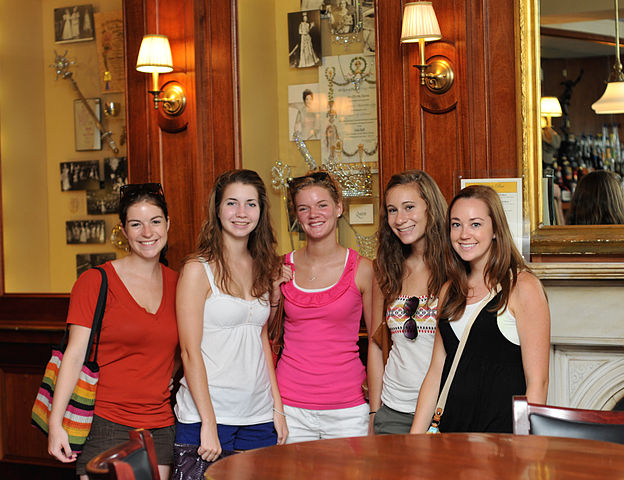# Optimise The Students

Probability Level 5A literature examination has $n$ candidates and 5 passage-based questions. For each question, each candidate is given a positive integer score which is at most 7. (They are copying the IMO. However, note here that the graders are nice. They refuse to give $0$ marks.) It turns out that none of them were ideal literature students, since no pair of candidates had the same score on 2 different questions. Find the largest possible value of $n$.

×